Courses

# Olympiad Test: Dice - 1

## 10 Questions MCQ Test Mathematics Olympiad Class 7 | Olympiad Test: Dice - 1

Description
This mock test of Olympiad Test: Dice - 1 for Class 7 helps you for every Class 7 entrance exam. This contains 10 Multiple Choice Questions for Class 7 Olympiad Test: Dice - 1 (mcq) to study with solutions a complete question bank. The solved questions answers in this Olympiad Test: Dice - 1 quiz give you a good mix of easy questions and tough questions. Class 7 students definitely take this Olympiad Test: Dice - 1 exercise for a better result in the exam. You can find other Olympiad Test: Dice - 1 extra questions, long questions & short questions for Class 7 on EduRev as well by searching above.
QUESTION: 1

### The positions of a cube are shown below, Which letter will be on the face opposite to face with ‘A’?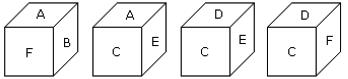Solution:

The letters of the adjacent faces to the face with letter A are B, F, C and E. Hence D is the letter of the face opposite to the face with letter (A).

QUESTION: 2

### Two positions of a dice are shown below. When 3 points are at the bottom, how many points will be at the top?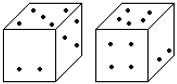Solution:

According to the rule (2), when 3 points are at the bottom then 4 points will be at the top.

QUESTION: 3

### Observe the dots on the dice (one to six dots) in the following figures. How many dots are contained on the face opposite to the face containing four dots?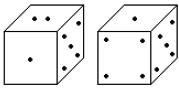Solution:

Here one of the two common faces (5) is in the same position, then according to the rule (2), the remaining face with the 4 dots will be opposite to face with dots 2.

QUESTION: 4

How many points will be on the face opposite to the face which contains 3 points?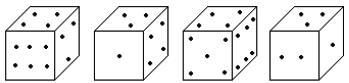Solution:

The adjacent faces to the face which 3 points have 2, 1, 4 and 6 points. Hence on the face which is opposite to the face which contains 3 points, there will be 5 points.

QUESTION: 5

When the digit 5 is on the bottom, which number will be on its upper surface?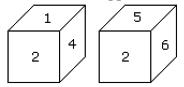Solution:

According to the rule (3), common faces with number 2 are in same positions. Hence when the digit 5 is on the bottom, 1 will on the upper surface.

QUESTION: 6

From the four positions of a dice given below, find the colour which is opposite to yellow?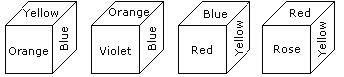Solution:

The colours adjacent to yellow are orange, blue, red and rose. Hence violet will be opposite to yellow.

QUESTION: 7

Two positions of a cubical block are shown. When 5 is at the top, which number will be at bottom?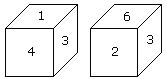Solution:

In these 2 positions one common face with number 3 is in the same position. Hence according to rule (3), 1 is opposite to 6 and 4 is opposite to 2. Therefore 5 is opposite to 3.

QUESTION: 8

Here 4 positions of a cube are shown. Which sign will be opposite to ‘+’?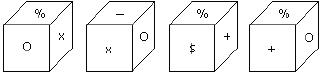Solution:

From position I and III common face with % is in the same position. Hence according to rule (3) opposite is x.

QUESTION: 9

Two positions of a dice are shown below. How many points will be on the top when 2 points are at the bottom?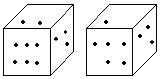Solution:

In these 2 positions of a dice, one common face having points 3 is in the same position. Hence according to rule (3), there will be 4 points on the required face.

QUESTION: 10

Here two positions of a dice are shown. If there are two dots in the bottom, then how many dots will be on the top?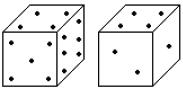Solution:

Here the common faces with 4 dots are in the same positions.
Hence 2 will be opposite to 5.